# Applied Math with VEXcode VR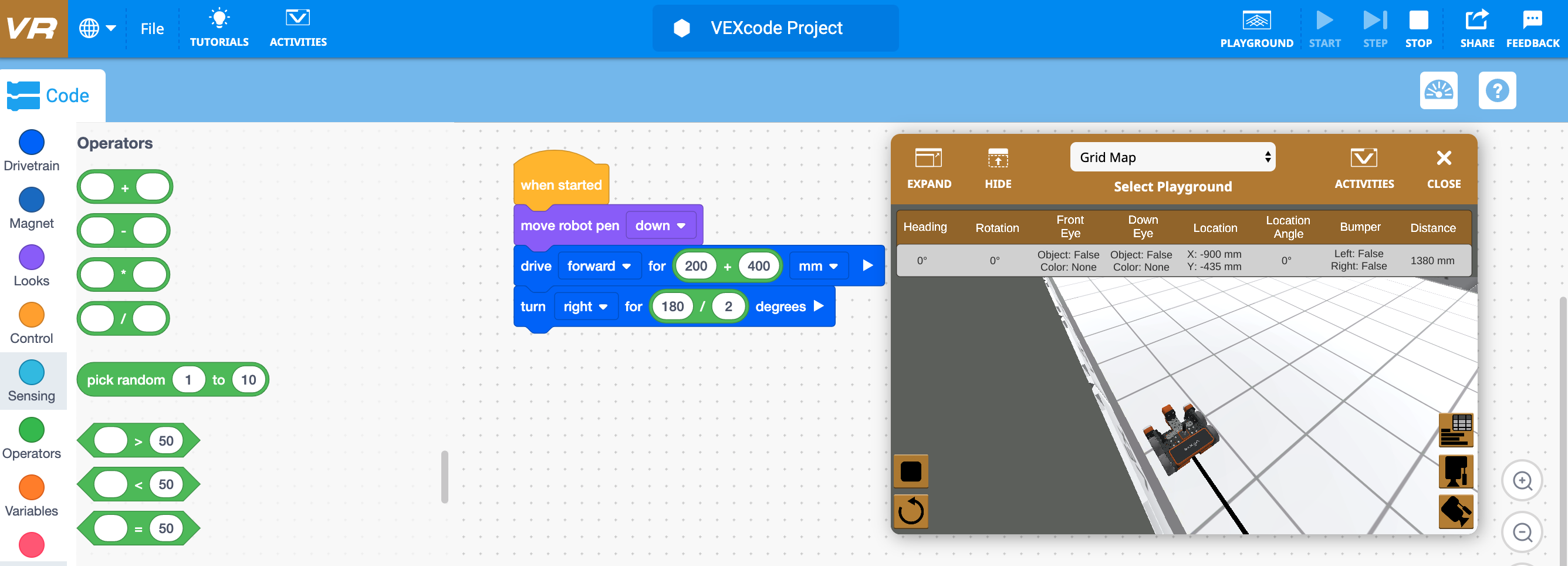VEXcode VR can be used to teach and practice many different math concepts such as order of operations, solving equations, solving right triangles, using the Pythagorean Theorem, categorizing shapes, and many others.

## Operator Blocks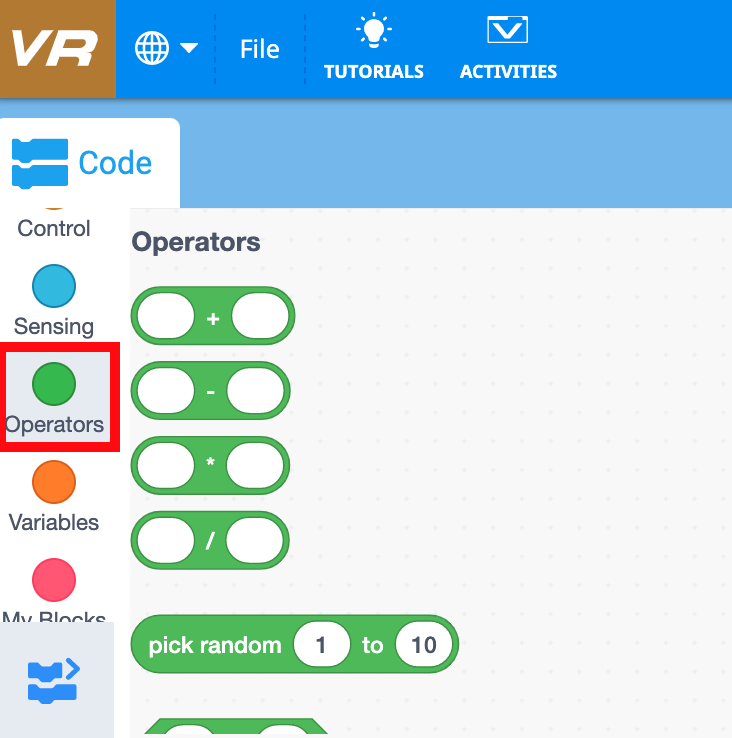Operator blocks are part of the Operators category in VEXcode VR. These blocks fall under the category of Reporter blocks, so they report values from variables, sensors, or calculations. For more information on Reporter blocks, view the Block Shapes and Meaning article.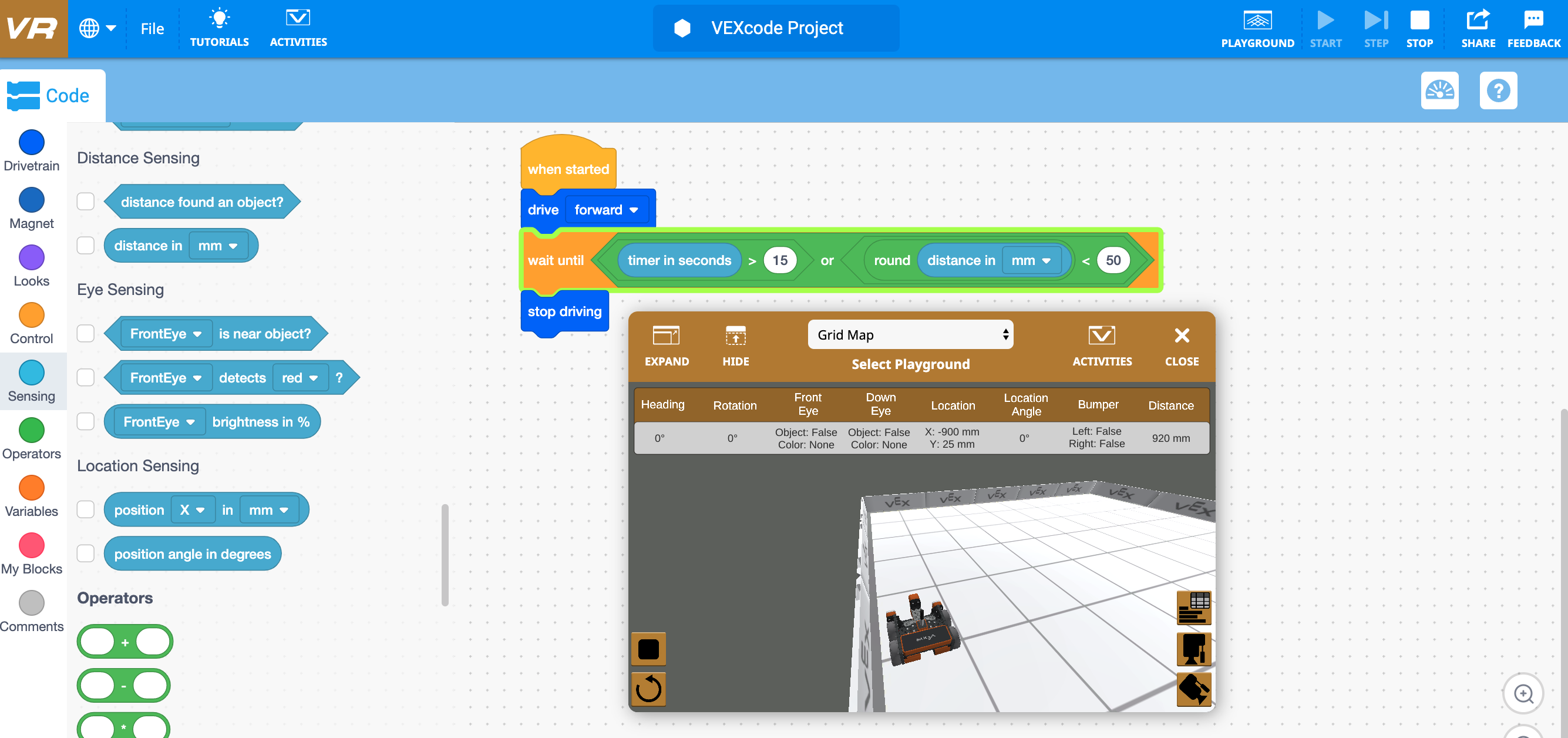Operator blocks can be used to determine calculations such as:

• Basic operations (addition, subtraction, multiplication, division)
• Rounding
• Absolute value
• Trigonometric functions (sine, cosine, tangent, arcsine, arccosine, arctangent)
• Logarithms
• Determine inequalities
• Utilize conjunctions (and), disjunctions (or,) and negations (not) that are used in discrete mathematics.

## Using the Monitor Window and Monitor Console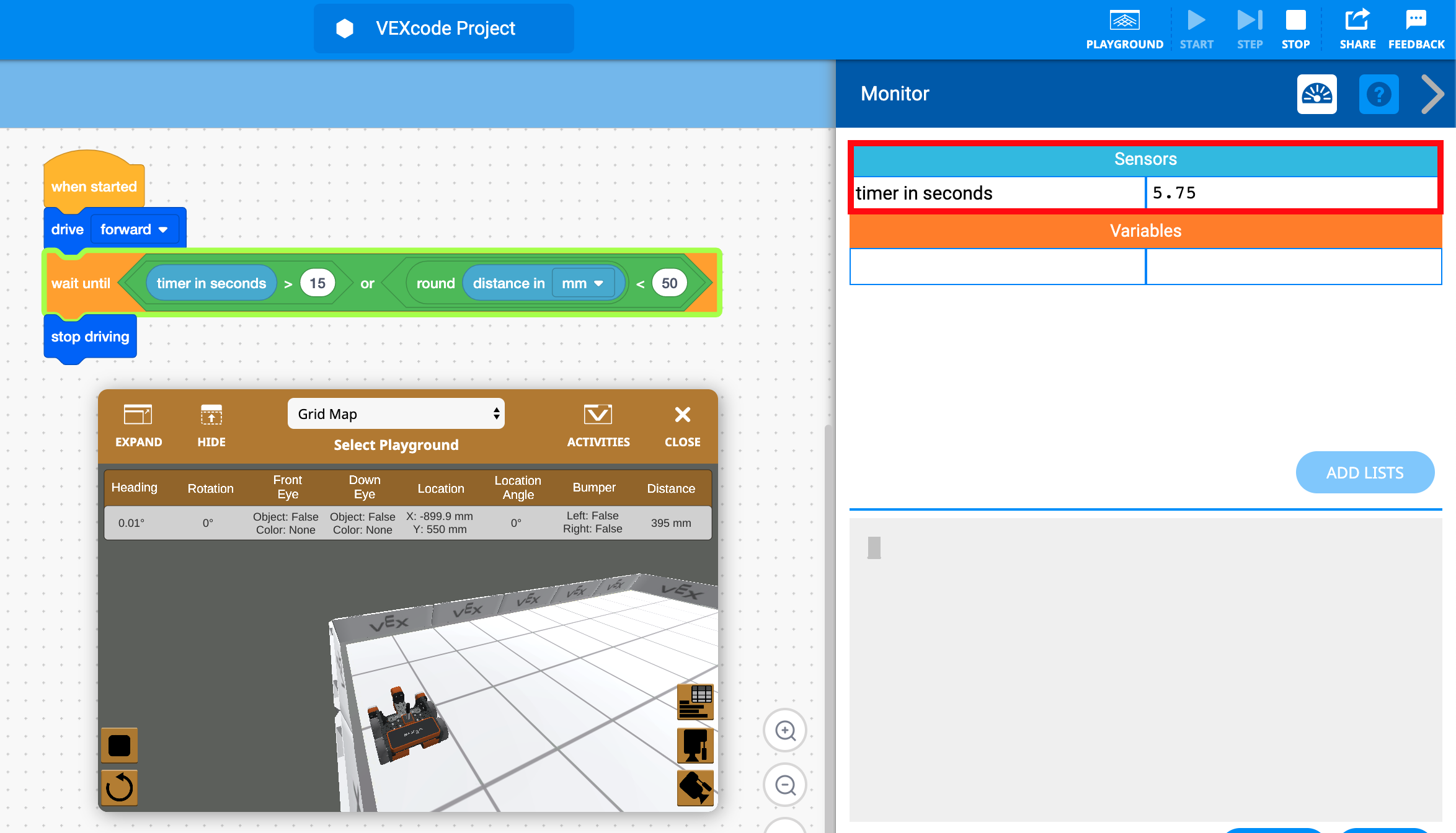The Monitor Window and Monitor Console can be used to display a message, report sensor values, or to collect data, creating user-readable outputs from VEXcode VR projects. This can be helpful when determining mathematical calculations.

For example, in the following project, being able to see the current value of the timer in seconds in the Monitor Window can allow the user to see which of the statements in the disjunction (the Or block) will cause the conditional to be true. Since the VR Robot will reach the wall before the 15 second threshold, the other condition in the Or block that the VR Robot will be less than 50 mm from the wall, will be true.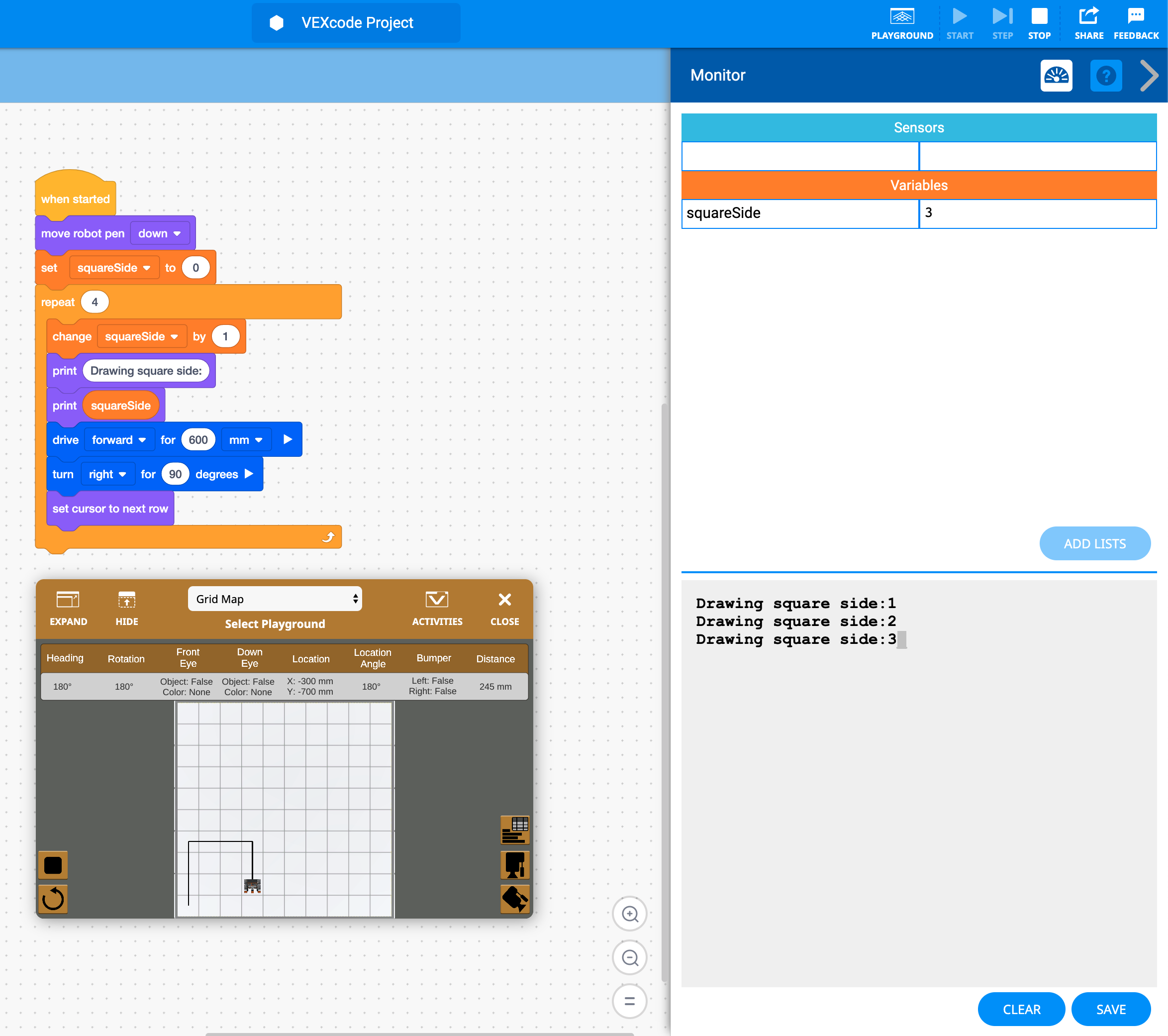The Print Console can also be used to view discrete moments in a project, such as viewing different sides being drawn to categorize shapes or printing calculations.

In the following example, the Monitor Console or the Monitor Window could be used to view which side of the square the VR Robot is actively drawing. This helps the user to better categorize shapes by their number of sides (triangle, quadrilateral, pentagon, hexagon, etc…).

## Pythagorean Theorem Example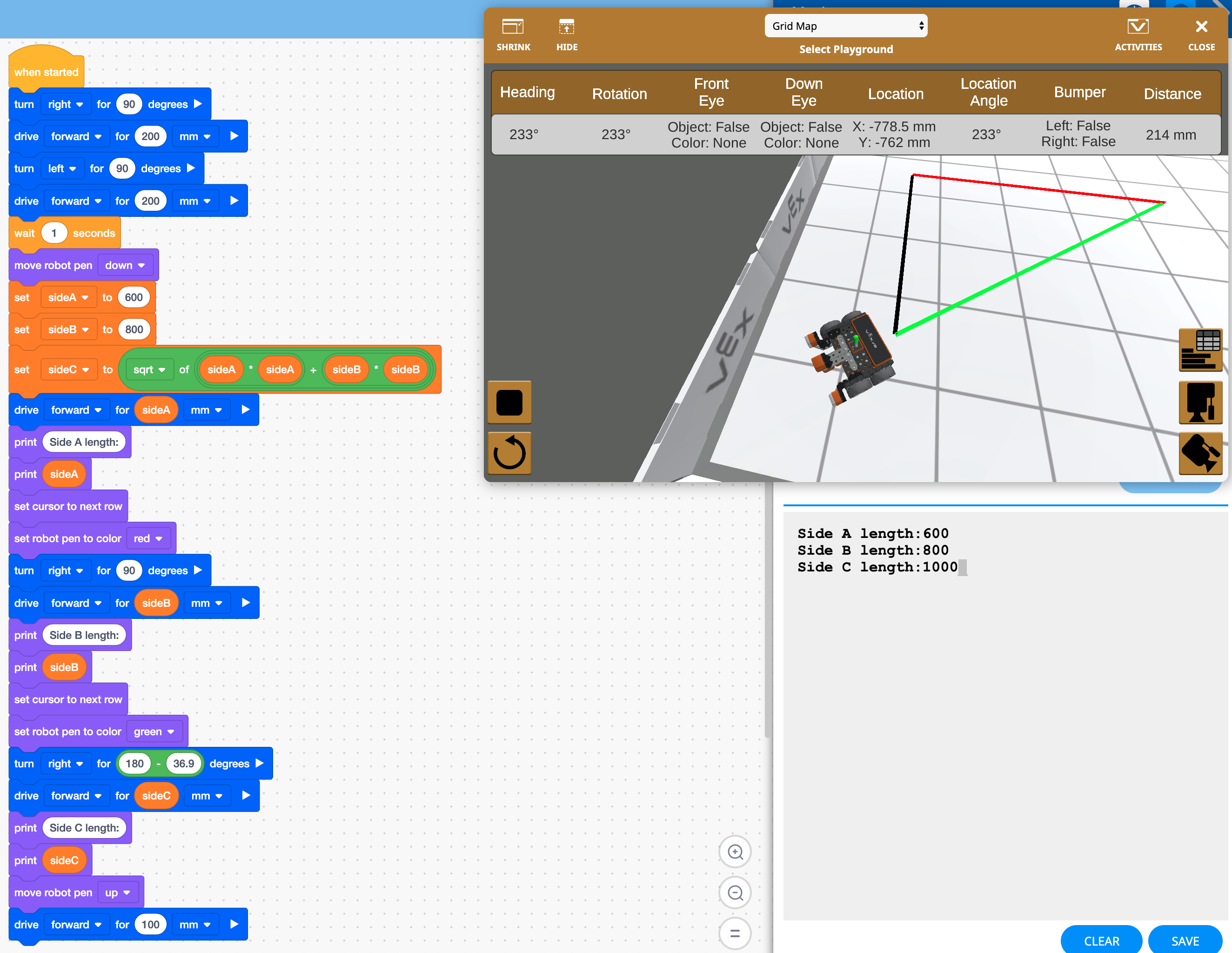In the following example, the VR Robot will solve for the third side of a Pythagorean Triple using the Pythagorean Theorem. The Pythagorean Theorem is used to find a missing side of a right triangle. The formula is the following:

Pythagorean Theorem: a2 + b2 = c2

In this example, the two sides given are 600 and 800 mm. The user is to calculate the third side using blocks from the Operators category. Some known properties of a Pythagorean Triple are that the sides are in a 3:4:5 ratio, and the three interior angle measurements are approximately 90, 36.9, and 53.1 degrees.

The project will use variables and operator blocks to calculate the missing side. The Monitor Console will be used to observe the length of all three sides, once they are calculated. This allows the user to see the value of the third side as it is calculated.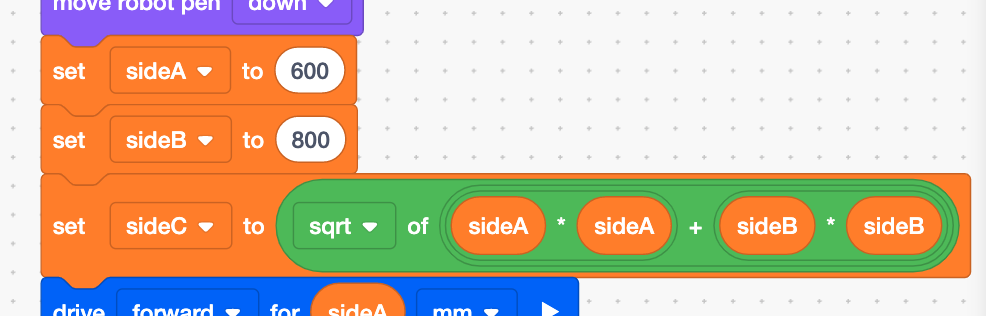Note how the formula is created in the project using the variable and operator blocks: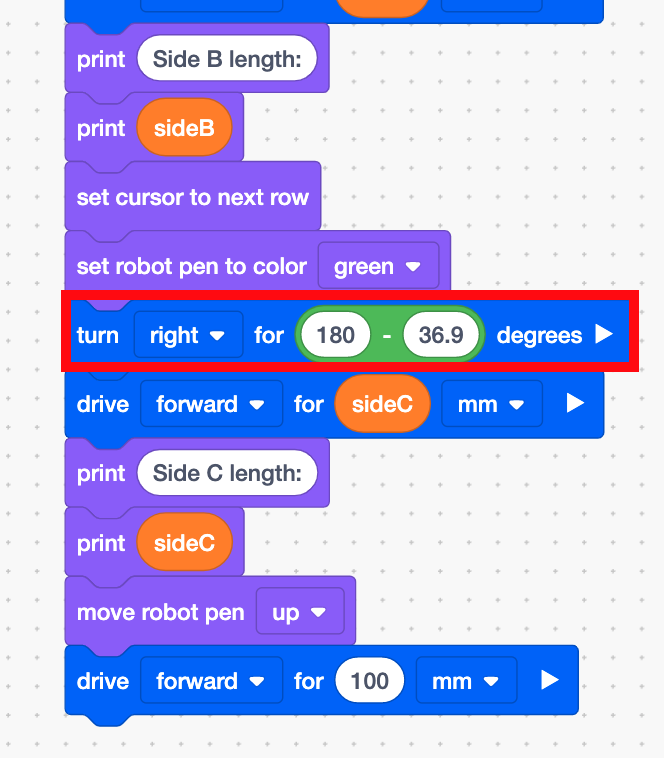Also note that the robot will have to turn the exterior angle of 143.1 degrees and not the interior angle of 36.9 degrees because of the way that the robot is facing after it draws side B.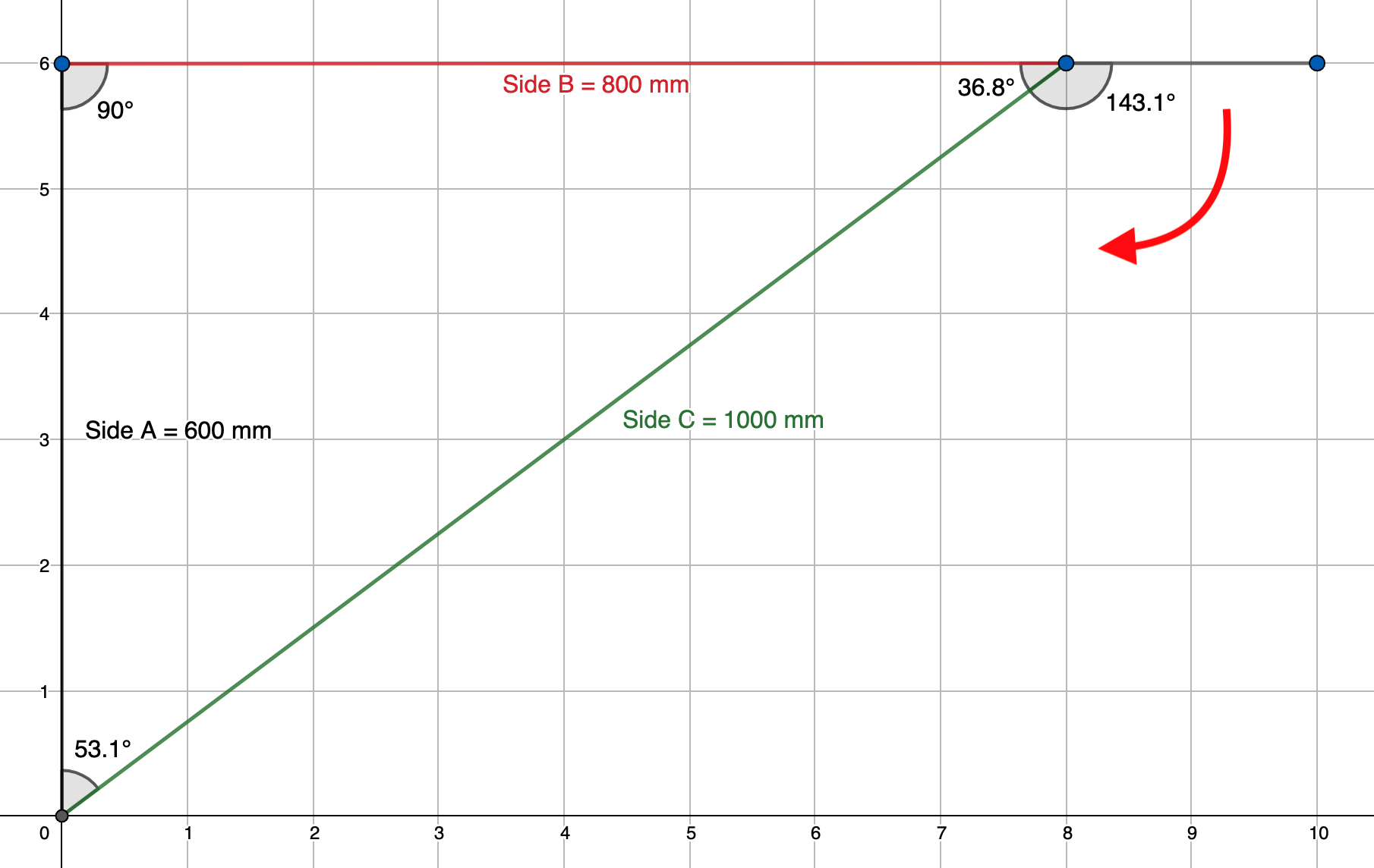36.9 degrees is the interior angle of the triangle, but the VR Robot will have to turn the value of the exterior angle in order to draw the triangle properly.

For more information, help, and tips, check out the many resources at VEX Professional Development Plus

Last Updated: